# Important questions on rotation for JEE Main/Advanced, Competitive examination

In this page we have Important questions on rotation for JEE Main/Advanced, Competitive examination . Hope you like them and do not forget to like , social share and comment at the end of the page.
A horizontal turn table carries a gun at point X and rotates with initial angular velocity ω0  about its vertical geometric axis. The Gun fires a bullet of mass m with tangential muzzle velocity v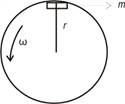I0 is the moment of inertia of the turntable and gun excluding bullet
Question 1 Find the initial angular momentum of the system about the axis of rotation
a)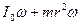b)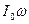c)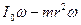d) None of these
Question 2 Find the final angular velocity of the turntable
a)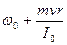b)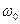c)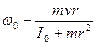d)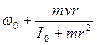A uniform rod (AB) of length L and mass 2M rest on smooth horizontal surface. A point mass M moving horizontally at right angles to the rod with initial velocity v collides with one end (A) and sticks to it
Question 3 Find the velocity of the center of mass of the system after the collision
a) v/3
b) v/2
c) v/4
d) 3v/4
Question 4 Find the angular velocity of the rod after the collision
a)v/L
b) v/2L
c) 2v/L
d) 3v/4L
Question 5
Match the column
Column A -> Velocity of the various part of the rod
Column B -> Their values in terms of v
Column A
P) Velocity of the end A
Q) Velocity of the end B
R) Velocity of the point C which is at distance 2L/3 from point A
S) Velocity of point D which is at distance L/2 from A
Column B
U) -v/3
V) 2v/3
W) 0
X) v/6

Question 6 which of the following will remain true in collision
a) Law of conservation of linear momentum
b) Law of conservation of angular momentum
c) Law of conservation of energy
d) None of these
Question 7 Find the total energy of the system after the collision
a)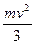b)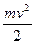c)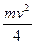d)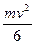Question 8 Which of the following is true?
a) The center of mass velocity before and after the collision will remain same
b) Moment of inertia about center of mass of the rod is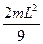c) Net torque and net force is zero on the system
d) None of these

Solutions